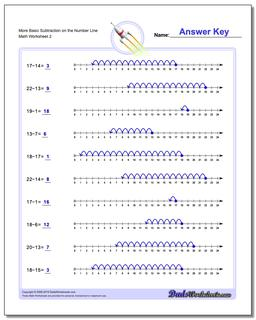One Dad. Four daughters. 9,161 worksheets... and counting!# More Basic Subtraction on the Number Line (Second Worksheet)

PropertyValue
DescriptionMore Basic Subtraction on the Number Line: These simple subtraction worksheets introduce subtraction concepts using the number Line. (Second Worksheet)
Resource TypeWorksheet